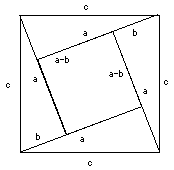# Disclaimer: The following material is being kept online for archival purposes.

## Although accurate at the time of publication, it is no longer being updated. The page may contain broken links or outdated information, and parts may not function in current web browsers.

 Site Map Math Index Glossary Timeline Questions & Answers Lesson Plans #M-7. Trigonometry

# (M-6) The Theorem of Pythagoras

 Pythagoras of Samos was a Greek philosopher who lived around 530 BC, mostly in the Greek colony of Crotona in southern Italy. According to tradition he was the first to prove the assertion (theorem) which today bears his name: If a triangle has sides of length (a,b,c), with sides (a,b) enclosing an angle of 90 degrees ("right angle"), then a2 + b2 = c2 A right angle can be defined here as the angle formed when two straight lines cross each other in such a way that all 4 angles produced are equal. The theorem also works the other way around: if the lengths of the three sides (a,b,c) of a triangle satisfy the above relation, then the angle between sides a and b must be of 90 degrees. For instance, a triangle with sides a = 3, b = 4, c = 5 (inches, feet, meters--whatever) is right-angled, because a2 + b2 = 32 + 42 = 9 + 16 = 25 = c2 Ancient Egyptian builders may have known the (3,4,5) triangle and used it (with measured rods or strings) to construct right angles; even today builders may still nail together boards of those lengths to help align a corner. Many proofs exist and the easiest ones are probably the ones based on algebra, using the elementary identities discussed in the preceding section, namely(a + b)2 = a2 + 2ab + b2 (recall that 2ab means 2 times a times b). For example 152 = (10 + 5)2    = 102 + (2)(10)(5) + 52    = 100 + 100 + 25    = 225 and (a – b) 2 = a2 – 2ab + b2 For example: 52 = (10 – 5)2    = 102 – (2)(10)(5) + 52    = 100 – 100 + 25    = 25 It is also necessary to know some simple areas: the area of a rectangle is (length) times (width), so the area of the one drawn above is ab. A diagonal cut divides it into two right-angled triangles with short sides a and b, and the area of such a triangle is therefore (1/2) ab.Now look at the square on the left constructed out of four (a,b,c) triangles. The length of each side is (a+b) and therefore the entire square has an area (a+b)2. However, the square can also be divided into four (a,b,c) triangles plus a square of side c in the middle (strictly speaking, we also ought to prove it is a square, but we will skip that). The area of each triangle, as shown earlier, is (1/2)ab, and the area of the square is c2. Since the big square is equal to the sum of all its parts (a + b) 2 = (4)(1/2)(a)(b) + c2 Using the identity for (a + b)2 and multiplying (4)(1/2) = 2 a2 + 2ab + b2 = 2ab + c2 Subtract 2ab from both sides and you are left with a2 + b2 = c2The same result can also be shown using a different square, of area c2. As the drawing on the right shows, that area can be divided into 4 triangles like the ones before, plus a small square of side (a– b). We get c2 = (4)(1/2)(a)(b) + (a– b) 2 = 2ab + (a2 – 2ab + b2) = a2 + b2        Q.E.D. Q.E.D. stands for "quod erat demonstrandum," Latin for "which was to be demonstrated," and in traditional geometry books those letters mark the end of a proof. The importance of the work of Pythagoras and of later masters of Greek geometry (especially Euclid) was not only in what they proved, but of the method they developed: start from some basic statements which are assumed to be valid ("axioms") and deduce by logic their more complicated consequences ("theorems"). Mathematics still follows the same pattern.    For a practical application of the Pythagoras theorem--deriving the distance to the horizon (neglecting atmospheric effects)--see here.   Optional:. The above is not the way Pythagoras and Euclid derived the theorem: their method was purely geometrical, not using numbers at all. For more about the way they did it, and more, see here.

Next Stop:   #M-7    Trigonometry: What is it good for?

Author and Curator:   Dr. David P. Stern
Mail to Dr.Stern:   stargaze("at" symbol)phy6.org .

Updated 25 November 2001, edited 27 October 2016.